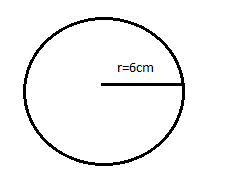# The diameter of a metallic sphere is $6cm$ . The sphere is melted and drawn into a wire of uniform circular cross-section. If the length of the wire is $36m$ , find the radius of its cross-section.$\left( a \right){\text{ 0}}{\text{.8cm}}$$\left( b \right){\text{ 0}}{\text{.5cm}}$$\left( c \right){\text{ 0}}{\text{.3cm}}$$\left( d \right){\text{ 0}}{\text{.1cm}}$Verified
176.4k+ views
Hint: Here in this question we will use the concept of volume of a sphere and the concept of volume of a cylinder. As the sphere is getting melted so a wire which will be cylindrical will be formed and the volume will be equal. By using this information we will be able to solve this problem.

Formula used:
The volume of a sphere is given by
${V_s} = \dfrac{4}{3}\pi {r^3}$
And the volume of a cylinder is given by,
${V_c} = \pi {R^2}h$
Here,
${V_c}$ , will be equal to the volume of a cylinder.
${V_s}$ , will be equal to the volume of a sphere.
$r$ , will be the radius and
$h$ , will be the height.

Here in this question it is given that the wire is in the shape of a cylinder. As we can see that the sphere is melted and then the cylindrical wire is formed from it.Hence, the volume will get equals.
So, we have the diameter which is equal to $6cm$ . Therefore, the radius will be equal to $3cm$ and the length of wire will be equals to $36m$ and will be termed as $h$
$\Rightarrow h = 3600cm$
Therefore, from the discussion, the volume of the sphere is equal to the volume of the wire.
Hence, on equating both the formulas, we get
$\Rightarrow \dfrac{4}{3}\pi {r^3} = \pi {R^2}h$
Like term will cancel out each other and from this on substituting the values, we get the equation as
$\Rightarrow \dfrac{4}{3}{\left( 3 \right)^3} = {R^2} \times 3600$
On solving the above equation, we get the equation as
$\Rightarrow {R^2} = \dfrac{1}{{100}}$
On removing the square, we get
$\Rightarrow R = \dfrac{1}{{10}}$
So on removing the fraction, it will be written as $0.1cm$ .
Therefore, the radius of the cross-section wire will be equal to $0.1cm$ .
Hence, the option $\left( d \right)$ is correct.

Note: For solving this type of question we just need the formula and analyzing the values given to us and the value we have to find. With carefulness, we can solve this type of question easily. But yes, we have to memorize those formulas. Also we should check the units while solving the question.